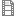# Hirzebruch-Riemann-Roch as a categorical trace (Recordings)

Posted in
Speaker:
Dennis Gaitsgory
Affiliation:
Harvard University
Date:
Tue, 2017-01-10 12:30 - Fri, 2017-01-13 14:30
Location:
MPIM Lecture Hall

Let X be a smooth proper scheme over a field of characteristic 0, and let E be a vector bundle on X.  The classical Hirzebruch-Riemann-Roch says that the Euler characteristic of the cohomology H^*(X,E) equals \int_X  ch(E) Td(X).
Thus, HRR is an equality of numbers, i.e., elements of a set.  In these talks,
we will explain a proof of HRR that uses the hierarchy
{2-categories} -> {1-categories} -> {Vector spaces} -> {Numbers}.
I.e., the origin of HRR will be 2-categorical.  The procedure by which we
go down from 2-categories to numbers is that of *categorical trace*.

However, in order to carry out our program, we will need to venture into
the world of higher categories: the 2-category we will be working with
consists of DG-categories, the latter being higher categorical objects.
And the process of calculation of the categorical trace will involve derived
algebraic geometry: the key geometric player will be the self-intersection
of the diagonal of X, a.k.a. the inertia (derived) scheme of X.

So, this series of talks can be regarded as providing a motivation for studying
higher category theory and derived algebraic geometry: we will use them
in order to prove an equality of numbers. That said, we will try to make these
talks self-contained, and so some necessary background will be supplied.

Video:Hirzebruch-Riemann-Roch1.mp4HirzebruchRiemannRoch2-1.mp4HirzebruchRiemannRoch2-2.mp4Hirzebruch-Riemann-Roch3.mp4
 © MPI f. Mathematik, Bonn Impressum & Datenschutz